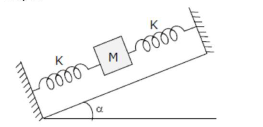# In the given figure, a body of mass $M$ is held between two massless springs, on a smooth inclined plane.Question:

In the given figure, a body of mass $M$ is held between two massless springs, on a smooth inclined plane. The free ends of the springs are attached to firm supports. If each spring has spring constant $\mathrm{k}$, the frequency of oscillation of given body is :1. (1)

$\frac{1}{2 \pi} \sqrt{\frac{2 k}{\operatorname{Mg} \sin \alpha}}$

2. (2) $\frac{1}{2 \pi} \sqrt{\frac{\mathrm{k}}{\mathrm{Mg} \sin \alpha}}$

3. (3) $\frac{1}{2 \pi} \sqrt{\frac{2 \mathrm{k}}{\mathrm{M}}}$

4. (4) $\frac{1}{2 \pi} \sqrt{\frac{\mathrm{k}}{2 \mathrm{M}}}$

Correct Option: 1

Solution:

(1)